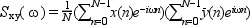Scilab Home page | Wiki | Bug tracker | Forge | Mailing list archives | ATOMS | File exchange
Change language to: Français - Português - 日本語

See the recommended documentation of this function

Scilab manual >> Signal Processing > pspect

# pspect

two sided cross-spectral estimate between 2 discrete time signals using the Welch's average periodogram method.

### Calling Sequence

```[sm [,cwp]]=pspect(sec_step,sec_leng,wtype,x [,y] [,wpar])
[sm [,cwp]]=pspect(sec_step,sec_leng,wtype,nx [,ny] [,wpar])```

### Arguments

x

vector, the time-domain samples of the first signal.

y

vector, the time-domain samples of the second signal. If `y` is omitted it is supposed to be equal to `x` (auto-correlation). If it is present, it must have the same numer of element than `x`.

nx

a scalar : the number of samples in the `x` signal. In this case the segments of the `x` signal are loaded by a user defined function named `getx` (see below).

ny

a scalar : the number of samples in the `y` signal. In this case the segments of the y signal are loaded by a user defined function named `gety` (see below). If present `ny` must be equal to `nx`.

sec_step

offset of each data window. The overlap `D`is given by sec_leng -sec_step```. if sec_step==sec_leng/2``` 50% overlap is made. The overlap

sec_leng

Number of points of the window.

wtype

The window type

• `'re'`: rectangular

• `'tr'`: triangular

• `'hm'`: Hamming

• `'hn'` : Hanning

• `'kr'`: Kaiser,in this case the wpar argument must be given

• `'ch'`: Chebyshev, in this case the wpar argument must be given

wpar

optional parameters for ```Kaiser and Chebyshev windows:```

• 'kr': ```wpar must be a strictly positive number```

• 'ch': `wpar` must be a 2 element vector `[main_lobe_width,side_lobe_height]with``0<main_lobe_width<.5`, and `side_lobe_height>0`

sm

Two sided power spectral estimate in the interval `[0,1]` of the normalized frequencies. It is a row array with `sec_len` elements . The array is real in case of auto-correlation and complex in case of cross-correlation.

The associated normalized frequencies array is `linspace(0,1,sec_len)`.

cwp

unspecified Chebyshev window parameter in case of Chebyshev windowing, or an empty matrix.

### Description

Computes the cross-spectrum estimate of two signals `x` and `y` if both are given and the auto-spectral estimate of `x` otherwise. Spectral estimate obtained using the modified periodogram method.

The cross spectrum of two signal `x` and `y` is defined to beThe modified periodogram method of spectral estimation repeatedly calculates the periodogram of windowed sub-sections of the data containes in `x` and `y` . These periodograms are then averaged together and normalized by an appropriate constant to obtain the final spectral estimate. It is the averaging process which reduces the variance in the estimate.

For batch processing, the `x` and `y` data may be read segment by segment using the `getx`and `gety` user defined functions. These functions have the following calling sequence:

`xk=getx(ns,offset)` and `yk=gety(ns,offset)` where `ns` is the segment size and `offset` is the index of the first element of the segment in the full signal.

### Reference

Oppenheim, A.V., and R.W. Schafer. Discrete-Time Signal Processing, Upper Saddle River, NJ: Prentice-Hall, 1999

### Examples

```rand('normal');rand('seed',0);
x=rand(1:1024-33+1);

//make low-pass filter with eqfir
nf=33;bedge=[0 .1;.125 .5];des=[1 0];wate=[1 1];
h=eqfir(nf,bedge,des,wate);

//filter white data to obtain colored data
h1=[h 0*ones(1:max(size(x))-1)];
x1=[x 0*ones(1:max(size(h))-1)];
hf=fft(h1,-1); xf=fft(x1,-1);y=real(fft(hf.*xf,1));

//plot magnitude of filter
h2=[h 0*ones(1:968)];hf2=fft(h2,-1);hf2=real(hf2.*conj(hf2));
hsize=max(size(hf2));fr=(1:hsize)/hsize;plot(fr,log(hf2));

//pspect example
sm=pspect(100,200,'tr',y);smsize=max(size(sm));fr=(1:smsize)/smsize;
plot(fr,log(sm));
rand('unif');```

C. Bunks INRIA